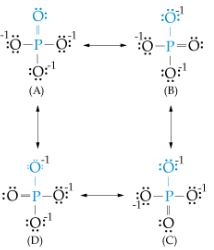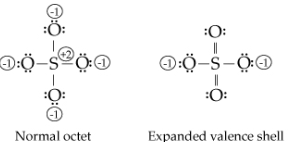# Problem: Part C. The bond order for a molecule is the number of bonds between a pair of atoms. For example, for the oxygen molecule, O = O , the bond order is two; for the hydrogen molecule, H − H , the bond order is one. Bond order indicates the strength of the bond.°Calculate the average bond order for a P−O bond (such as the one shown in blue) in a phosphate ion. Express your answer numerically as a decimal or improper fraction.For example, consider the two Lewis structures for the sulfate ion (SO42−):In the normal octet structure, sulfur has a +2 formal charge and all four oxygen atoms have a − 1 formal charge. Because sulfur belongs to the third period, it shows an expanded octet that minimizes the formal charge on each of the atoms. Thus in the expanded Lewis structure for a sulfate ion, the sulfur atom has 12 valence electrons, and the formal charge on the sulfur atom gets reduced to 0 by forming two sulfur-oxygen double bonds. The formal charge on two oxygen atoms also gets reduced to 0.

###### FREE Expert SolutionView Complete Written Solution
###### Problem Details

Part C. The bond order for a molecule is the number of bonds between a pair of atoms. For example, for the oxygen molecule, O = O , the bond order is two; for the hydrogen molecule, H − H , the bond order is one. Bond order indicates the strength of the bond.

°Calculate the average bond order for a P−O bond (such as the one shown in blue) in a phosphate ion.